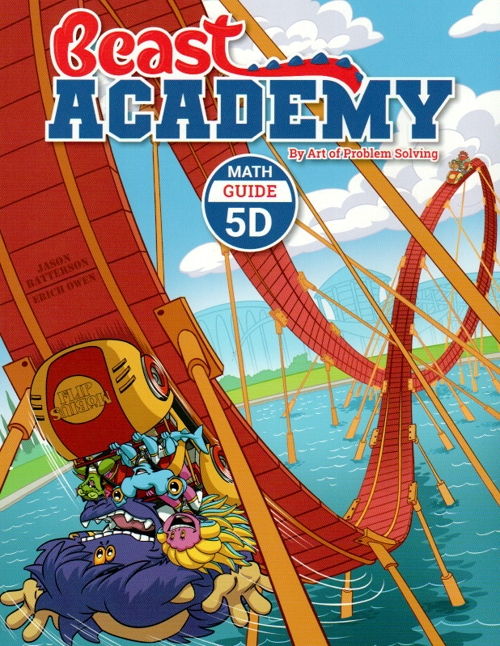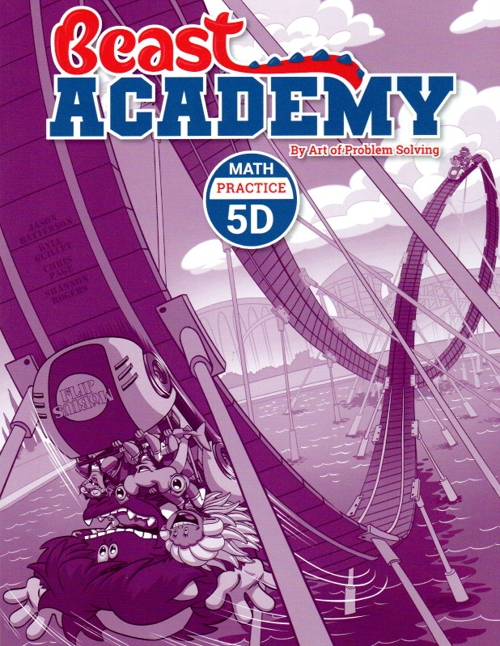Art of Problem Solving Beast Academy 5D Guide and Practice \$30 : Percents : Converting among fractions, decimals, and percents, estimation, proportions, and percent change. Square Roots : Estimation, comparison, and the Pythagorean theorem. Exponents : Multiplying and dividing powers, negative exponents, scientific notation, raising a power to a power, and solving equations.